# Circuit's RMS output is linearly proportional to temperature over wide range

## Analog Devices LT1001

It is known that the p-n diode can be the basis of an accurate thermometer for cryogenic temperatures up to about 200 °C. A constant current is maintained through the diode and the voltage across it gives an indication of the temperature. The magnitude of the constant current is typically chosen small to minimize diode self heating. The diode voltage falls linearly with temperature making it necessary to have an operational amplifier conditioning circuit for direct readout. Output calibration is achieved using a voltage reference.

In this design idea the concept of the p-n diode driven at constant current is extrapolated, potentially eliminating voltage references and op amps. The result is a unique implementation of a simple but highly accurate temperature proportional circuit. Interesting applications are also suggested. The operation of the circuit is based on a switched scheme that allows two alternate constant currents to flow through the diode. The method is well grounded in the mathematics but the sombre details are omitted in this article.

The starting point is Shockley’s diode equation: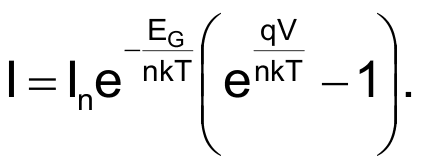The equation relates the current (I) flowing through a p-n diode to the voltage (V) across it, the temperature (T) and the ideality factor (n) of the semiconducting material of the diode. Boltzmann’s constant is, and the electronic charge is q. The term In is a non-temperature dependent part of the reverse saturation current of the diode. In a reported experiment  a silicon 1N4148 diode is alternately driven first at current I1, and then at constant current I2, with the changeover occurring at a temperature T.

Figure 1 shows the effect of the two currents on the diode voltage as it is cooled in a water bath from temperature T0. In principle, the segments AB and CD extrapolated backwards meet at absolute zero (–273 °C). However, impurity and other effects in the diode limit circuit usefulness to about –200 °C.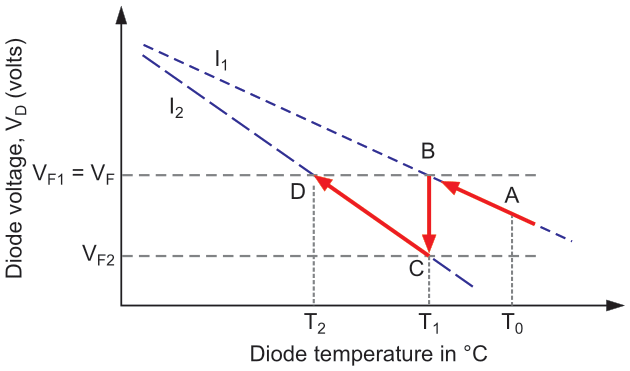Figure 1: Operating principle of the direct RMS readout thermometer.

At temperature T the use of two sequentially switched currents gives the data pair: (T, I1, V1), and (T, I2, V2). Putting the pair into Shockley’s equation allows the difference voltage ΔV = V1(T) – V2(T) to be found easily in terms of the ideality factor as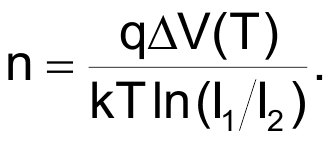The ideality factor is typically constant for a given diode, being 1.9 for the 1N4148 diode . Observed circuit predictability is better for silicon diodes than germanium diodes. In the last equation, the terms n, q/kT and ln(I1/I2) being constant, one can rewrite using a new constant b:

ΔV(T) = bT, where T is in Kelvin

Therefore the voltage difference at a given temperature T is directly proportional to the temperature provided that the currents are switched in ratio at the measurement temperature. The switchover time is shorter than the time over which the temperature can change. For higher measurement accuracy the voltage difference ΔV at the temperature of interest should be large. Figure 1 suggests a large ratio I1/I2, but self heating could arise if I1 is made too large. Figure 2 shows an innovative, switched constant-current mirror made up of transistors Q1 and Q2 with the output current mirrored in D1.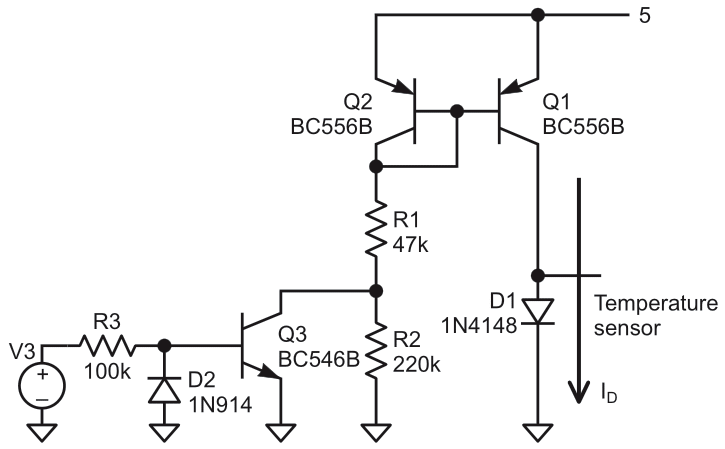Figure 2: Switched constant-current source.

In Figure 2 the load resistor of Q2 is switched into or out of the collector by an extra transistor, Q3 driven by a clock source V3 having 50% duty cycle. Thus the load resistance variably has the value R1 when V3 = 5 V and (R1 + R2) when V3 = 0 V. The values shown give two mirror currents of 20.14 µA or 111.38 µA. Substituting all the constants into the voltage difference equation gives

ΔV(T) = 2.8 × 10– 4T.

This equation predicts outputs of ΔV = 20.5 mV at –200 °C, 83.4 mV at 25 °C and 132.0 mV at 200 °C. Amplification using op amps can boost the output to the required level.

In the final circuit the clock source can be a 555-astable with near 50% duty-cycle, or an embedded controller PWM output. A design was tested at 10 kHz. The diode voltage switches between V1 and V2 and therefore has an inherent dc level that can filtered using a simple RC-network with a low cut-off frequency, e.g., 2 Hz if needed.

Filtering guarantees square-wave output with negligible peak level attenuation without introducing frequency dependence. The output can then be readout at “OUT1” in Figure 3 on a true RMS digital voltmeter in AC mode.

The upper operating frequency limit is that at which the diode switching response becomes sluggish. Several readout enhancements are possible. For example, connecting a unity-gain detector or precision full-wave rectifier  to the filter output allows an output that is positive with temperature proportional peak and RMS values at “OUT2” in Figure 3.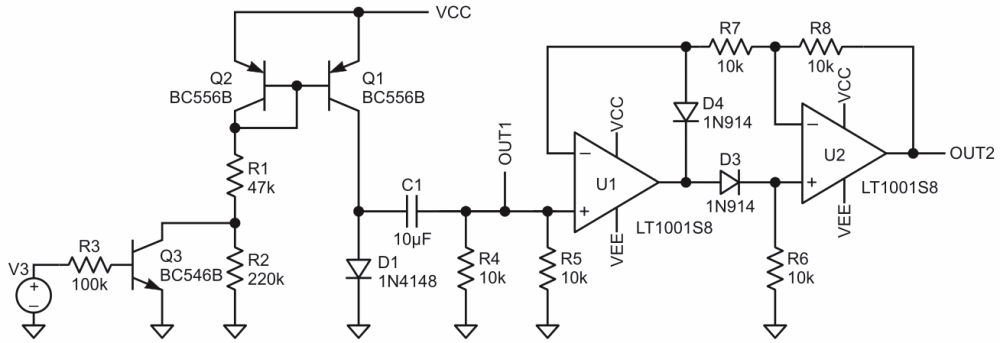Figure 3: Functional circuit with RMS output directly proportional to temperature.

Possible applications of the circuit are direct read-out ultra-linear, wide-range thermometers, closed-loop temperature control, and temperature data acquisition. Interesting applications are amplitude modulation of a high-frequency carrier by the filtered output waveform. Frequency modulation by temperature is also possible if the RC-filter is removed and the diode DC centered voltage first buffered and then applied to the tuning varactor in a linear VCO. The frequency deviation is then directly proportional to the temperature.

## References

1. R O Ocaya and P V C Luhanga 2011 Eur. J. Phys. 32 1155
2. Jones D and Stitt, Precision Absolute Value circuits, SBOA068, Burr-Brown

EDN